Open In App
Related Articles

# How to draw 2D Heatmap using Matplotlib in python?

In this article, we will explain about plotting heatmaps using the matplotlib library. A heatmap is a great tool for visualizing data across the surface. It highlights data that have a higher or lower concentration in the data distribution.

## Heatmap

A 2-D Heatmap is a data visualization tool that helps to represent the magnitude of the matrix in form of a colored table. In Python, we can plot 2-D Heatmaps using the Matplotlib and Seaborn packages. There are different methods to plot 2-D Heatmaps, some of which are discussed below.

## Use Cases For Heatmaps

As we know the Heatmap is just a colored representation of a matrix. However, heatmap has a very large use case. We can use heatmaps for the following purpose.

•  It is used to see the correlation between columns of a dataset where we can use a darker color for columns having a high correlation.
•  We can also use heatmaps for plotting various time series and finance-related data where the Y-axis will be the month and X-axis will be the year and the element of the heatmap will be our data.

## Basic Heatmap Using Python Matplotlib Library

### Create a 12×12 Heatmap with Random data using Matplotlib

Here we will plot the heatmap using matplotlib.pyplot.imshow() function.

Syntax: matplotlib.pyplot.imshow(X, cmap=None, alpha=None)

• X :- this is input data matrix which is to be displayed
• cmap :- Colormap we use t dispay the heatmap
• alpha :- it specifies the opacity or transpiracy of the heatmap

## Python3

 `# Program to plot 2-D Heat map``# using matplotlib.pyplot.imshow() method``import` `numpy as np``import` `matplotlib.pyplot as plt` `data ``=` `np.random.random(( ``12` `, ``12` `))``plt.imshow( data )` `plt.title( ``"2-D Heat Map"` `)``plt.show()`

Output: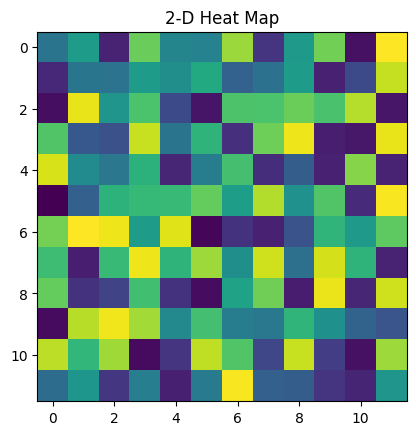Hetamap of random 12×12 matrix

### Choosing Different Colormaps in Heatmap Using Matplotlib

We can choose different colors for Heatmap using the cmap parameter. cmap can help us in making our heatmap more informative.

## Python3

 `# Program to plot 2-D Heat map``# using matplotlib.pyplot.imshow() method``import` `numpy as np``import` `matplotlib.pyplot as plt` `data ``=` `np.random.random((``12``, ``12``))``plt.imshow(data, cmap``=``'autumn'``)` `plt.title(``"Heatmap with different color"``)``plt.show()`

Output: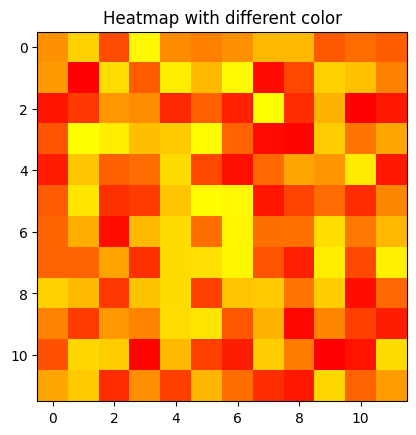heatmap with camp parameter

### Adding Colorbar to Heatmap Using Matplotlib

we can add a colorbar to the heatmap using plt.colorbar(). colorbar shows the weight of color relatively between a certain range.

## Python3

 `data ``=` `np.random.random((``12``, ``12``))``plt.imshow(data, cmap``=``'autumn'``, interpolation``=``'nearest'``)` `# Add colorbar``plt.colorbar()` `plt.title(``"Heatmap with color bar"``)``plt.show()`

Output: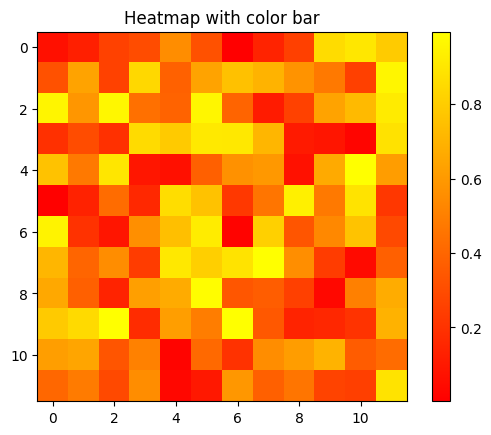Heatmap with colorbar scale

## Customized Heatmap Using Matplotlib Library

we can customize this heatmap using different functions and parameters to make it more informative and beautiful. we will use plt.annotate()  to annotate values in the heatmap. Also, we will use colors library to customize the color of the heatmap.

## Python3

 `import` `matplotlib.colors as colors` `# Generate random data``data ``=` `np.random.randint(``0``, ``100``, size``=``(``8``, ``8``))` `# Create a custom color map``# with blue and green colors``colors_list ``=` `[``'#0099ff'``, ``'#33cc33'``]``cmap ``=` `colors.ListedColormap(colors_list)` `# Plot the heatmap with custom colors and annotations``plt.imshow(data, cmap``=``cmap, vmin``=``0``,\``           ``vmax``=``100``, extent``=``[``0``, ``8``, ``0``, ``8``])``for` `i ``in` `range``(``8``):``    ``for` `j ``in` `range``(``8``):``        ``plt.annotate(``str``(data[i][j]), xy``=``(j``+``0.5``, i``+``0.5``),``                     ``ha``=``'center'``, va``=``'center'``, color``=``'white'``)` `# Add colorbar``cbar ``=` `plt.colorbar(ticks``=``[``0``, ``50``, ``100``])``cbar.ax.set_yticklabels([``'Low'``, ``'Medium'``, ``'High'``])` `# Set plot title and axis labels``plt.title(``"Customized heatmap with annotations"``)``plt.xlabel(``"X-axis"``)``plt.ylabel(``"Y-axis"``)` `# Display the plot``plt.show()`

Output: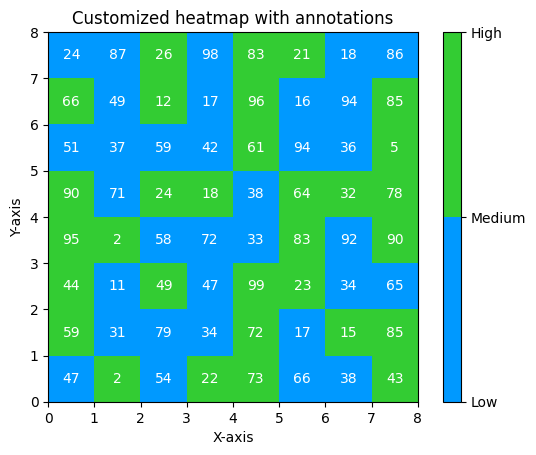advance customized heatmap using matplotlib library

## Plotting Correlation Matrix of a Dataset Using Heatmap

Next, we will use a heatmap to plot the correlation between columns of the dataset. We will use correlation to find the relation between columns of the dataset. We can download the dataset from here

## Python3

 `import` `pandas as pd``import` `matplotlib.pyplot as plt``from` `matplotlib ``import` `colors` `df ``=` `pd.read_csv(``"gold_price_data.csv"``)` `# Calculate correlation between columns``corr_matrix ``=` `df.corr()` `# Create a custom color``# map with blue and green colors``colors_list ``=` `[``'#FF5733'``, ``'#FFC300'``]``cmap ``=` `colors.ListedColormap(colors_list)` `# Plot the heatmap with custom colors and annotations``plt.imshow(corr_matrix, cmap``=``cmap, vmin``=``0``\``           ``, vmax``=``1``, extent``=``[``0``, ``5``, ``0``, ``5``])``for` `i ``in` `range``(``5``):``    ``for` `j ``in` `range``(``5``):``        ``plt.annotate(``str``(``round``(corr_matrix.values[i][j], ``2``)),\``                     ``xy``=``(j``+``0.25``, i``+``0.7``),``                     ``ha``=``'center'``, va``=``'center'``, color``=``'white'``)` `# Add colorbar``cbar ``=` `plt.colorbar(ticks``=``[``0``, ``0.5``, ``1``])``cbar.ax.set_yticklabels([``'Low'``, ``'Medium'``, ``'High'``])` `# Set plot title and axis labels``plt.title(``"Correlation Matrix Of The Dataset"``)``plt.xlabel(``"Features"``)``plt.ylabel(``"Features"``)` `# Set tick labels``plt.xticks(``range``(``len``(corr_matrix.columns)),\``           ``corr_matrix.columns, rotation``=``90``)``plt.yticks(``range``(``len``(corr_matrix.columns)),``           ``corr_matrix.columns)` `# Display the plot``plt.show()`

output: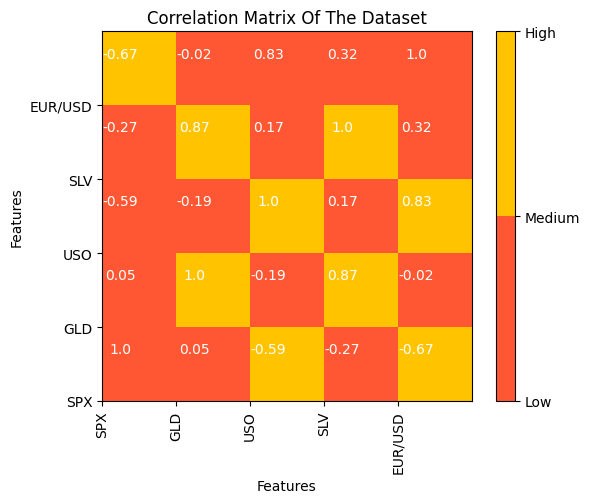Correlation Matrix of the Dataset

## Heatmap Using Seaborn Library

We can also use the Seaborn library to plot heatmaps even plotting heatmaps using Seaborn is comparatively easier than the matplotlib library. To plot the heatmap using Seaborn we will use sns.heatmap() function from the Seaborn library.

## Python3

 `# importing the modules``import` `numpy as np``import` `seaborn as sns``import` `matplotlib.pyplot as plt``  ` `# generating 2-D 10x10 matrix of random numbers``# from 1 to 100``data ``=` `np.random.randint(low``=``1``,``                         ``high``=``100``,``                         ``size``=``(``10``, ``10``))``  ` `# plotting the heatmap``hm ``=` `sns.heatmap(data``=``data,``                ``annot``=``True``)``  ` `# displaying the plotted heatmap``plt.show()`

Output: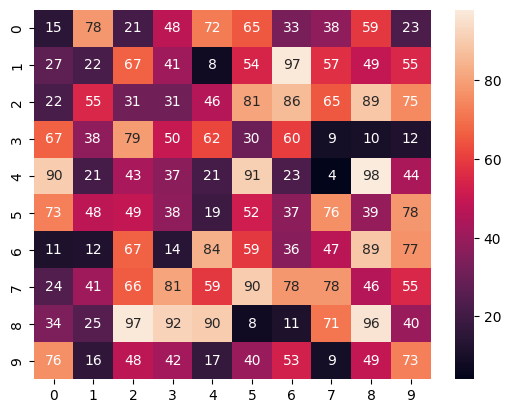Heatmap using seaborn